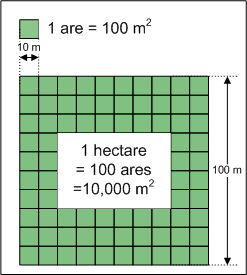# Definition of Hectare (ha)A hectare is a metric unit of area. It is equal to $10,000$ square metres.

One hectare is equal to $100$ ares, and one are is equal to $100 \text{ m}^2$.

Hectares are most often used to measure the area of land. A square with a side-length of 100 metres has an area of 1 hectare.

We use the symbol ha for hectares.

In case your grandparents are wondering, $1 \text{ ha}$ is approximately $2.47$ acres.

### Description

The aim of this dictionary is to provide definitions to common mathematical terms. Students learn a new math skill every week at school, sometimes just before they start a new skill, if they want to look at what a specific term means, this is where this dictionary will become handy and a go-to guide for a student.

### Audience

Year 1 to Year 12 students

### Learning Objectives

Learn common math terms starting with letter H

Author: Subject Coach
You must be logged in as Student to ask a Question.